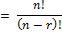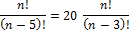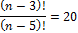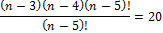## Practice Questions – Permutation and Combination – 1

1) Find the value of 9P3.

(a) 504

(b) 309

(c) 405

(d) 600

(a) 504

Explanation:

9P3 = 9!/6!

Because, nPr= (9 ×8 ×7 ×6!)/6!

= 9 × 8 × 7 = 504

2) Find the value of n, if nP5 = 20 nP3.

(a) 5

(b) 8

(c) 6

(d) 4

(b) 8

Explanation:

nP5 = 20 nP3(n – 3)(n – 4) = 20

n = 8 (n = -1 is inadmissible)

3) How many numbers greater than 5000 can be formed with the digits, 3, 5, 7, 8, 9 no digit being repeated?

(a) 216

(b) 126

(c) 512

(d) 252

(a) 216

Explanation:

 4 4 3 2

= 4 × 4 × 3 × 2 = 96

Thousands place cannot assume 3 since required numbers are greater than 5000.

 5 4 3 2 1

= 5 × 4 × 3 × 2 × 1 = 120

Total required numbers = 96 + 120 = 216.

4) How many 6 digit telephone numbers can be constructed with the digits 0, 1, 2, 3, 4, 5, 6, 7, 8, 9 if each number starts with 35 and no digit appears more than once?

(a) 1600

(b) 1680

(c) 900000

(d) 9000

(b) 1680

Explanation:

 8 7 6 5

= 8 × 7 × 6 × 5 = 1680

3 and 5 have been already used, so we have 8 digits for thousands place, then 7 digits for hundreds place, 6 digits for tens digit and remaining 5 digits for unit place.

5) How many 6 digit numbers can be formed from the digits 1, 2, 4, 5, 6, 7 (no digit being repeated) which are divisible by 5?

(a) 555

(b) 156

(c) 120

(d) None of these

(c) 120

Explanation:

A number is divisible by 5 only if its unit digit is either 5 or 0. So we fix 5 as unit digit and then we fill up the remaining places.

 5 4 3 2 1

= 5 × 4 × 3 × 2 × 1 = 120

Hence, required number is 120.

6) How many even numbers greater than 300 can be formed with the digits 1, 2, 3, 4, 5 no digit being repeated?

(a) 111

(b) 600

(c) 900

(d) None of these

(a) 111

Explanation:

Case 1

 3 3 1

= 3 × 3 × 1 = 9

When 2 is fixed at unit place, we have 3, 4 and 5 i.e., 3 digits for hundreds place and remaining 3 digits (out of 5) for the tens place.

 2 3 1

= 2 × 3 × 1 = 6

When 4 is fixed at unit place, we have 3 and 5 i.e., only two digits for hundreds place and remaining 3 digits for tens place.

Case 2

 4 3 2 2

= 48

Case 3

 4 3 2 1 2

= 48

5 digit numbers = 48

Thus there are total 15 + 48 + 48 = 111 even numbers greater than 300.

7) In how many ways the letters of the word RAINBOW be arranged?

(a) 5040

(b) 4050

(c) 3040

(d) 8040

(a) 5040

Explanation:

There are 7 letters in the word RAINBOW and each letter is used only once. So all the 7 letters can be arranged in 7! Ways.

7! = 7 × 6 × 5 × 4 × 3 × 2 × 1 = 5040

8) In the word RAINBOW, how many words begin with R?

(a) 720

(b) 360

(c) 1440

(d) None of these

(a) 720

Explanation:

If we fix R as initial letter, then we have to arrange only 6 remaining letters.

Hence required number of permutations = 6!

= 6 × 5 × 4 × 3 × 2 × 1 = 720

9) In the word RAINBOW, how many words begin with R and ends with W?

(a) 120

(b) 240

(c) 180

(d) 360

(a) 120

Explanation:

If we fix R and W as the first and last letters then we have to arrange only 5 remaining letters which can be arranged in 5! = 120 ways.

10) In the word RAINBOW, how many words are there in which I and O are never together?

(a) 1440

(b) 720

(c) 3600

(d) None of these

(c) 3600

Explanation:

Number of permutations when I and O are together

= 2! × 6! = 1440

Total number of permutations = 7! = 5040

Therefore, number of permutations when I and O are not together

= 5040 – 1440 = 3600